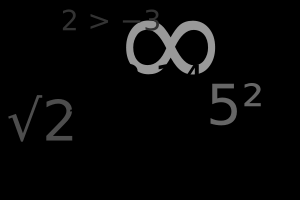# If I had 6 tickets to theatre, four of which are in the front row, and 3 of those tickets are selected at random, how do I work out the likelihood of those 3 tickets being front row tickets?

What you are looking for is the probability of of those 3 tickets being front row tickets. Probability is the maths of chance. A probability is a number that tells you how likely, or probable, something is to happen. This most commonly used example of probability is of rolling a die. Each number has a 1 in 6 chance of being rolled, making a probability of .16666 rec.The probability of an event equals the number of ways an event can occur divided by the total number of possible outcomes. If we go back to our die, we might asks, what is the probability of each outcome? What is the probability of rolling an even number? Of rolling an odd number?

In the mathematical sense, 'outcome' means the result of one roll of the die - we know one number will be rolled and in that instance, it didn't matter which. If we roll the die, learning whether the number rolled is odd or even is known as the 'outcome,' as there is more than one category of options.

The Odds are Even for the Odd Numbers

We know that out of the 6 sides of the die, there are 3 odd numbers on a die (1, 3 and 5) and 3 even numbers (2, 4 and 6). The probability of rolling odd is the same as that of rolling even - 3 in 6 (or put more simply 1 in 2). 3 divide 6 is 0.5. There is a 0.5 probability of rolling an odd number, the same as for rolling an even number; or, expressed as a percentage, a 50% probability.

In your example, the probability of any of the 6 tickets being front row tickets is 4 in 6, or 0.6666 rec. or 66.7% (to one decimal place). As for the rest of the sum, here's a film to give you a few ideas:

thanked the writer.